# Engineering Mechanics - KOP: Work and Energy

### Exercise :: KOP: Work and Energy - General Questions

• KOP: Work and Energy - General Questions
11.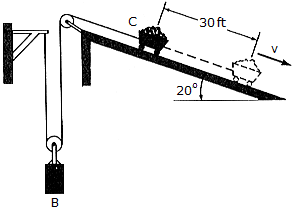The car C and its contents have a weight of 600 lb, whereas block B has a weight of 200 lb. If the car is released from rest, determine its speed when it travels 30 ft down the 20° incline.

 A. vC = 3.55 ft/s B. vC = 3.94 ft/s C. vC = 17.68 ft/s D. vC = 15.94 ft/s

Explanation:

No answer description available for this question. Let us discuss.

12.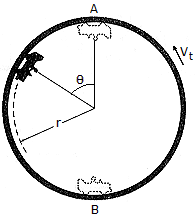The "flying car" is a ride at an amusement park, which consists of a car having wheels that roll along a track mounted on a drum. Motion of the car is created by applying the car's brake, thereby gripping the car to the track and allowing it to move with a speed of vt = 3m/s. If the rider applies the brake when going from B to A and then releases it at the top of the drum, A, so that the car coasts freely down along the track to B (=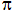rad), determine the speed of the car at B and the normal reaction which the drum exerts on the car at B. The rider and car have a total mass of m = 250 kg and the center of mass of the car and rider moves along a circular path of radius r = 8 m.

 A. vB = 12.88 m/s, NB = 2.45 kN B. vB = 12.88 m/s, NB = 7.64 kN C. vB = 17.97 m/s, NB = 12.54 kN D. vB = 17.97 m/s, NB = 7.64 kN

Explanation:

No answer description available for this question. Let us discuss.

13.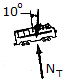An electric train car, having a mass of 25 Mg, travels up a 10° incline with a constant speed of 80 km/h. Determine the power required to overcome the force of gravity.

 A. P = 961 kW B. P = 346 kW C. P = 341 kW D. P = 946 kW

Explanation:

No answer description available for this question. Let us discuss.

14.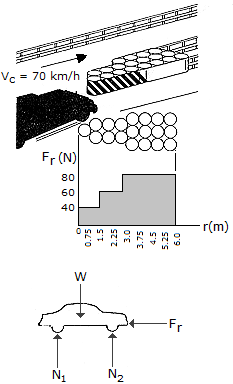A car, assumed to be rigid and having a mass of 800 kg, strikes a barrel-barrier installation without the driver applying the brakes. From experiments, the magnitude of the force of resistance Fr, created by deforming the barrels successively, is shown as a function of vehicle penetration. If the car strikes the barrier traveling at Vc = 70 km/h, determine approximately the distance s to which the car penetrates the barrier.

 A. s = 1.890 m B. s = 4.72 m C. s = 2.77 m D. s = 2.52 m

Explanation:

No answer description available for this question. Let us discuss.

15.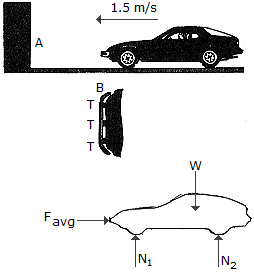A car is equipped with a bumper B designed to absorb collisions. The bumper is mounted to the car using pieces of flexible tubing T. Upon collision with a rigid barrier A, a constant horizontal force F is developed which causes a car deceleration of 3g = 29.43 m/s2 (the highest safe deceleration for a passenger without a seatbelt). If the car and passenger have a total mass of 1.5 Mg and the car is initially coasting with a speed of 1.5 m/s, compute the magnitude of F needed to stop the car and the deformation x of the bumper tubing.

 A. F = 44.1 kN, x = 38.2 mm B. F = 22.1 kN, x = 76.4 mm C. F = 22.1 kN, x = 38.2 mm D. F = 44.1 kN, x = 76.4 mm